Next: Fitting General Curves Up: Curve Fitting Previous: Curve Fitting

### Fitting Straight Lines

Suppose we have a set of N points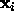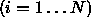and we wish to find the `best' straight line through them. To define `best' we need to give a quality of fit measure. Let us first choose an appropriate parameterization of the line;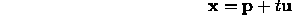where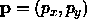is the closest approach of the line to the origin and thus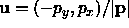is normal to the vector p. We choose this form as it gives both parameters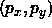the same units. It is simple to show that the normal distance of such a line to a pointis given by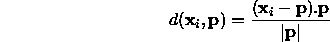If we assume a density distribution for this distance,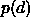, and that the points are independent samples from a distribution about the true line, we can construct a log likelihood function (see above)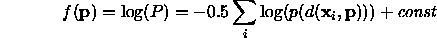which must be maximized. Ifis gaussian the problem can be solved by taking the line through the centroid of the N points parallel to the principle eigenvector of the covariance matrix of the data about the centroid. If, however, a non-gaussian distribution is used, we revert to using the optimization methods described above to choose the parameterswhich maximize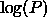. Typically we would use a local optimizer, obtaining a starting point by first solving with an assumed gaussian form for. If the functionis twice differentiable then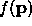is twice differentiable, and the Levenberg-Marquardt method can be used.

For instance, robust line fitting techniques accommodate outliers by assuming a distribution forwith longer tails than the gaussian . For instance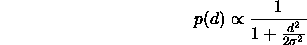or a gaussian with extended tails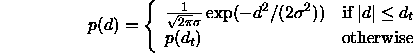Note that this latter is not strictly a probability density distribution since it does not have unit area, but this does not affect the parameter estimation. Note also that the latter is not differentiable at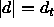, socan only be optimized using a method which does not require derivatives, such as Simplex or Powell's.

In any parameter estimation problem it is important to estimate the confidence region about the parameter estimates obtained. Ifis gaussian thenis a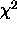function. The found parameters can be considered to be drawn from a multivariate normal distribution about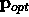(which achieve the optimum) with a covariance matrix given by the inverse of the Hessian of f evaluated at. Confidence ellipsoids aboutcan be drawn up at suitable levels using this covariance matrix . Ifis not gaussian then more detailed analytic calculations or a Monte Carlo simulation are required to obtain the confidence limits.

The approach given above makes the assumption that measurement errors in the data points are equal in all directions. If this is not the case the merit function must be reformulated accordingly.Next: Fitting General Curves Up: Curve Fitting Previous: Curve Fitting

Bob Fisher
Fri Mar 28 14:12:50 GMT 1997